## Polygonal approximation of curves

www.kovalevsky.de, last update: October 26, 2011Let me know
what you think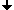The problem of polygonal approximationSchlesingers similarityThe split-and-merge methodThe sector methodImproved ector methodOptimal polygonal approximation

## The problem of polygonal approximation

Given is a digital curve C in a 2D image. We want to approximate C by a polygon which has as few as possible edges and is "similar" to C. One of the possible criterions of the similarity is the Hausdorff distance: Let S1 and S2 be two sets of points. Let us denote the distance between the points p and q as d(p,q). Then the following definitions hold: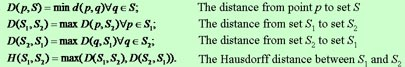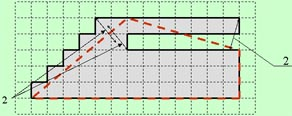Sample: All three small arrows have the length = 2. Here the Hausdorff distance between the black and the red curve is <2, however, the "true deviation" is much greater. Schlesinger has suggested a more adequate measure of the similarity of curves.

## Schlesingers similarity

Given are two digital curves C1 and C2 . Take m points along C1 and n points along C2. Thus one gets two ordered sets M1 and M2 of points. The smaller the distance between subsequent points the more precise the estimate of the similarity. Let us denote the distance between two points p and q as d(p,q).
Find the monotone map F: M1M2, where monotone means that for any two points p and q of M1 such that p > q also F(p) > F(q) holds. The sign " > " in "p > q" means that the point p follows the point q in the sequence M1. We look for the smallest value of the maximum distance between the corresponding points.
This value is the Schlesinger distance DS(M1, M2) = min( max(d(p, F(p) ));
Schlesinger has also suggested an efficient algorithm for computing the distance DS for any two digital curves. The value of DS for our example can be computed as follows. Let the black curve be B and the red one be R. They are subdivided into corresponding segments (a,b), (b,d), (d,f) and (f,a). Map each point of a segment of B onto the nearest point of the corresponding segment of R as shown in the figure.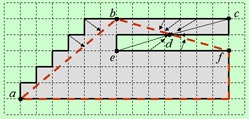The value of DS for the above example is defined by the length of (c,d) (or (e,d)) and is equal to 3.6 that is essentially greater than 2.

## Algorithms for the polygonal approximation: The split-and-merge method

The advantage of this method is that it is very simple to understand and to program. The idea is as follows. Given is the curve C and the maximum allowed distance ε from the points of C to the polygon. Take an arbitrary point P1 of C. Find the point P2 with the greatest distance from P1. Now the curve is subdivided into two segments: (P1, P2) and (P2, P1). Each segment (Pi, Pk) has its chord (at start two segments have the same chord). For each segment find the point Pm with the greatest distance d from the corresponding chord. If d>ε split the segment (Pi,Pk) into two segments (Pi,Pm) and (Pm,Pk) . Repeat this until d≤ε for all segments. The "split" phase is finished. Now check each pair of adjacent segments (Pi,Pm) and (Pm,Pk) whether the distance d of Pm to the chord (Pi,Pk) satisfies d≤ε. If so, merge the segments (Pi, Pm) and (Pm, Pk).

 An example with ε = 1.5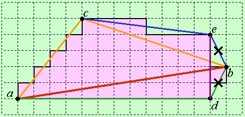Split phase: P1=a, P2=b, P3=c, P4=d, P5=e. Merge phase: (e,b) and (b,d) have been merged to (e, d).

## Algorithms for polygonal approximation: The sector method

The split-and-merge method is rather slow because each point must be tested many times, depending upon the number of splittings of the segment to which it belongs. This method minimizes the Hausdorff distance, not the Schlesinger distance.
An efficient method called the sector method was suggested in the 70th by Williams. The idea is as follows:
1) Start with some point P1 of the curve C. Test all subsequent points. For each point Pi with i>1 and d(P1, Pi)>ε draw a circle with the radius equal to the tolerance ε and draw two tangents from P1 to the circle. The space between the tangents compose a sector, while each straight line L through P1 lying in the sector has the property that the distance from the center of the circle to L is less than ε.
2) If the next point Pi (fig. P3 or P4) lies in the sector, then a new circle with its center at Pi and a sector S are constructed. The new sector is the intersection of S with the old one.
3) If the next point Pi (fig. P5) lies outside of the new sector, then there is no straight line L through P1 and Pi such that all points between P1 and Pi have a distance to L less than ε. Therefore Pi cannot serve as a polygon edge. The point before Pi (fig. P4) is then the last point of the current segment and the starting point of the next one.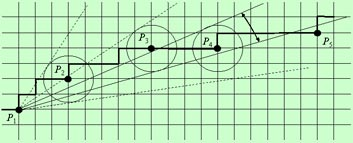## Algorithm of the improved sector method

The sector method is fast because it tests each point only once. However, it guaranties only that the Hausdorff distance between the curve and the polygon is less than the prescribed tolerance ε. The following additional condition for interrupting the current segment of the curve, that should be approximated by the current edge of the polygon, has been introduced. The point Pmax of the current segment, which has the greatest distance from the starting point of the segment, must be defined during the processing of the segment. If the projection of the actual point Pi onto the line (P1, Pmax) is less than |P1, Pmax|−ε then the point Pmax is the last point of the current segment and the starting point of the next one. This test guaranties that the sequence of the projections of the points of the curve onto the polygon is monotone and therefore the conditions for defining the Schlesingers distance are fulfilled.
In the following example red points are those at which the algorithm comes to the decision to interrupt the current segment. The tolerance ε = 1.5.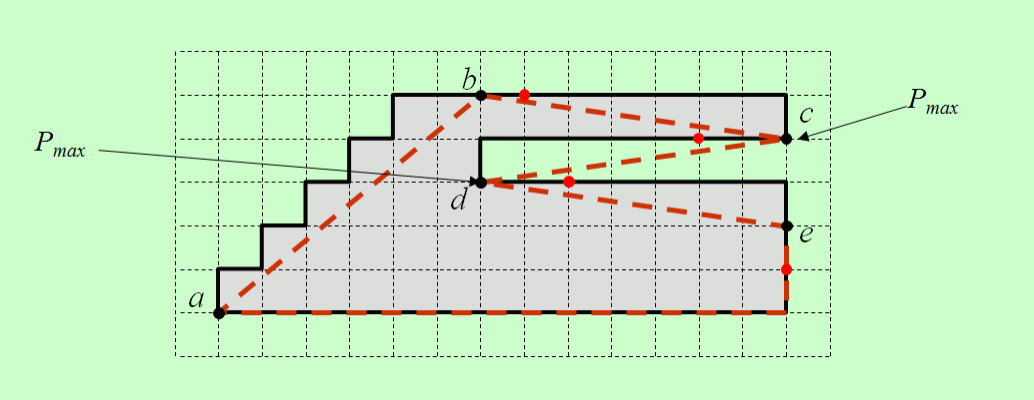## Optimal polygonal approximation

 An important drawback of the sector method is that it "cuts off" the vertices of right angles with sides parallel to the coordinate axes. The reason is that it only checks whether the distance of the given points from the polygon is less than the threshold.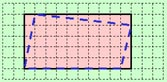A better method must also minimize the distance of the points from the polygon while retaining the number of polygon edges as small as possible. These contradictive requirements are expressed in the following minimization criterion: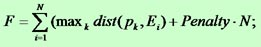Here is N the number of the polygon edges, "Penalty" is a predefined constant, dist(p,Ei) is the distance from the point pk belonging to the ith segment of the curve to the edge Ei. The maximum is with respect to all points of the ith segment.
The minimization problem can be solved by means of the dynamic programming in the following way. The curve (closed or open) is represented as a sequence of points p, k∈[1,M]. We allocate an array State[M] of structures, where M is the number of points. Each structure State[k] contains a float variable State[k].SUM and an integer variable State[k].iPopt.

 Let us draw the elements of State[ ] as a sequence of points (small disks) in the following graph: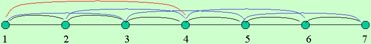The arcs represent possible polygon edges. Consider as an example (the figure below) the point 4 and all possible edges connecting it with some of the points with smaller numbers: the red arc connects the point 4 with the point 1, the blue one - with the point 2 and the black one - with point 3. The arc connecting the point k with the point i, i<k, defines a possible value of the criterion F: F(k)=F(i)+MaxDist(i,k)+Penalty, where MaxDist(i,k) is the maximum distance from the points of the segment (i, k) to the polygon edge (i,k). Thus, if we know the values of F(i) for all i<k, we can compute the values of F(k) corresponding to all arcs having their right ends at pk. We must find the smallest of these values, save it as State[k ].SUM and save the corresponding value of i as State[k ].iPopt. The value of State.SUM is 0. When starting from k=2 one can compute the optimal values for k=2, 3,..., M. After having reached the last point k=M one can find its previous optimal point with the index State[M].iPopt, then find its previous point etc. thus reconstructing the optimal sequence of the polygon vertices.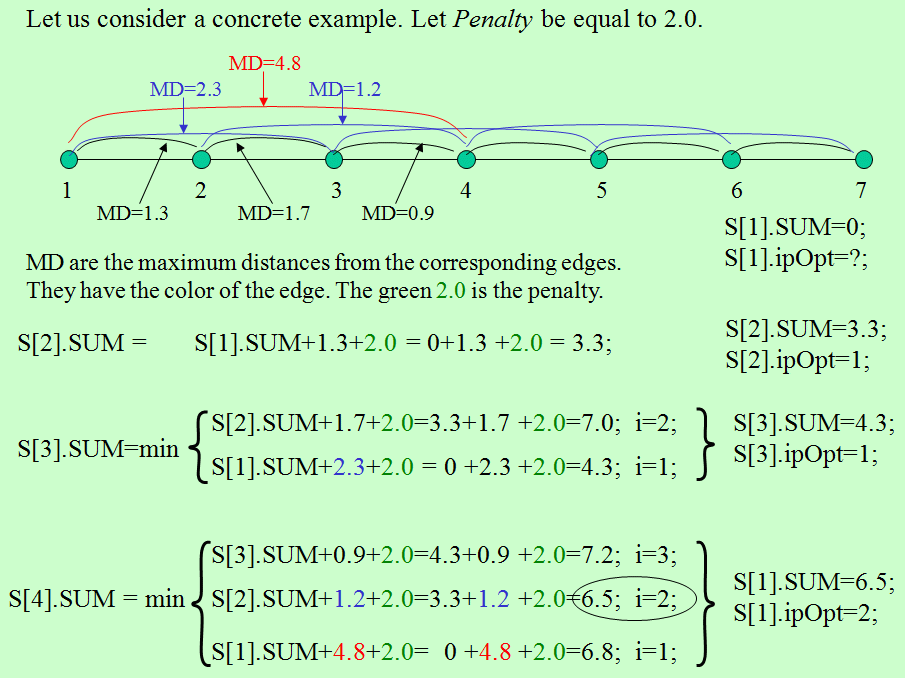This method brings the best results as to the precision of the approximation. However, it must be still improved as to its time consuming. One of the ways of doing this consists in subdividing the given curve into longest DSSs and applying the optimization method to the endpoints of the DSSs. However, this approach needs some additional research to find a fast method of estimating the maximum deviation of the points of a DSS from the line connecting its end points. It is also necessary to eliminate DSSs looking like the following two: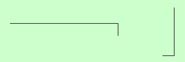since they will give rise to the above mentioned problem of "cutting off" the vertices of right angles. The version explained above brings the optimal solution for a fixed starting point of the polygon. The non-trivial, fast solution for the optimal starting point must be still developed. Thus, there is still some research work to be done to bring this method to perfection.